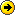# Nigeria Student Forum

Welcome, Guest: Join Nigeria Student Forum / Login / Trending Now / Recent Topics

Stats: 32,084 Members, 82,214 Topics, 16,480 Comments. Date: Sep 29 2020, 11:16 am

SPECIAL: Gain Admission Into 200 Level To Study In Any University Via IJMB | CAMBRIDGE | JUPEB | NO JAMB | LOW FEES | Call 07063085343, 08086347092 To Register!

## How To Calculate Grade Points Average (gpa) In Nigerian Polytechnics

(Go Down)
B.tech Marketing Management
GridCodes: Works even when there is no street name/house number. The GridCode you generate is a full fledged address that is smart as well. Download the GridCode App

Android: https://bit.ly/2U0hH4D

iOS Users: https://apple.co/2NaFgUD

The calculation of GPA in Nigeria polytechnics follows nearly the same procedure with that of Nigerian Universities. However Nigerian polytechnics make use of the following grading system in calculation of GPA and the awarding of Ordinary National Diploma (OND) and Higher National Diploma (HND):Assuming you offer the following courses and obtain scores that correspond to the following grades that lead to the distribution on the tables below. then you will your GPA as follows:First Semester OND1:

GPA = Total points/Total Credit Units = 44.00/15 = 2.9.

Therefore your first semester ND1 GPA = 2.9.
Second Semester OND1 GPA Calculation

Assuming you offer the following courses and obtain scores that correspond to the following grades that lead to the distribution on the tables below. then you will your GPA as follows:Second Semester GPA = Total points/Total Credit Units = 36.0/16 = 2.21875= 2.25.
Therefore your second semester ND1 GPA = 2.25.

Not, to calculate your cumulative grade point average (CGPA) for ND1 (first year), you add your ND1 first semester and second semester GPA and divide the result you obtain by two (2), i.e. the number of semester. This is simply finding the average.

Here it goes:
Your OND1 CGPA = (First Semester ND1 GPA + Second semester ND1 GPA) / 2 = (2.9 + 2.25) / 2.

CGPA = 5.15 / 2 = 2.575 = 2.58

The process is repeated for every semester result.
That should be all on how to calculate GPA (Grade Point Average) and CGPA (Cumulative Grade Point Average) in Nigerian Polytechnics. For addition or queries, use the comment box below.

2 LikesNgstudentforum.com Is The Next Big Hits Student Forum In Nigeria

E.g Seuncoded